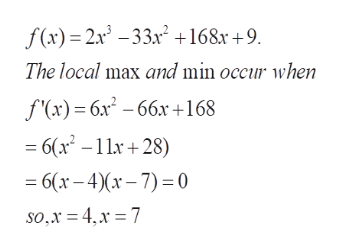# The function f(x)=2x3−33x2+168x+9 has one local minimum and one local maximum. Use a graph of the function to estimate these local extrema.This function has a local minimum at x =   with output value:   and a local maximum at x =   with output value:

Question
31 views

The function f(x)=2x3−33x2+168x+9 has one local minimum and one local maximum.
Use a graph of the function to estimate these local extrema.

This function has a local minimum at x =
with output value:

and a local maximum at x =
with output value:

check_circle

Step 1

To estimate (approximate calculation) the local maximum and local minimum of the given function y=f(x)

Step 2

Now we know the critical values are at x=4 and x=7. For further analysis graph the ...help_outlineImage Transcriptionclosef(x) 2x3-332 +168x +9 The local max and min occur when f(x) 6x2-66x168 - 6(x2 -11+28) 6(x-4)-7)0 so,x 4x 7 fullscreen

### Want to see the full answer?

See Solution

#### Want to see this answer and more?

Solutions are written by subject experts who are available 24/7. Questions are typically answered within 1 hour.*

See Solution
*Response times may vary by subject and question.
Tagged in

### Derivative